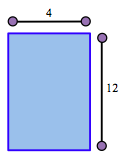### Home > ACC6 > Chapter 1 Unit 1 > Lesson CC1: 1.2.3 > Problem1-68

1-68.

Harry had a pile of $48$ pennies. He organized them into a rectangular array. It had exactly four rows with $12$ pennies in each row.

Draw diagrams to represent at least two other rectangular arrays Harry could use. Do you think there are more? Explain your thinking.Harry's penny rectangle

Try dividing $48$ by different numbers. Are any quotients whole numbers?

One possible rectangle is $1$ by $48$. What are some others?